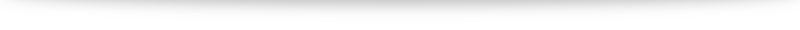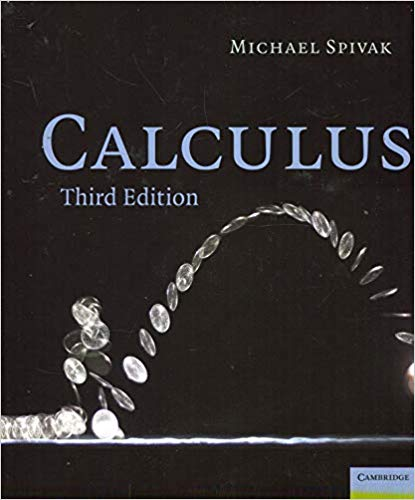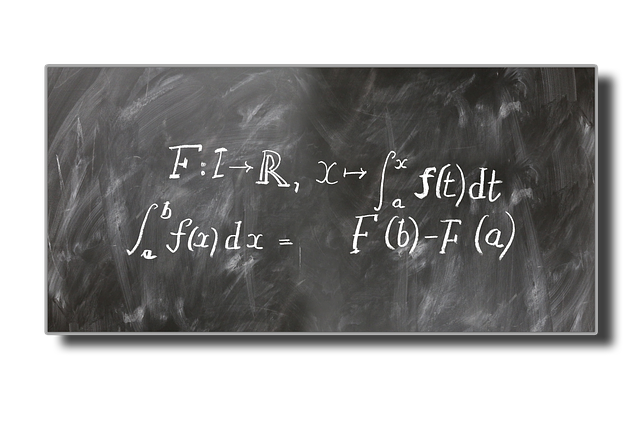# Chapter 1: Numbers, operations and axioms

### Numbers

Numbers are our first exposure to mathematics: yet what exactly a "number" is an interesting discussion I've had in philosophy classes. At least for "whole numbers", we typically find them pretty intuitive and it doesn't take a child much to be comfortable with numbers like "2" and "3" and learning how to operate on them via addition, "$2+3=5$" and multiplication "$2 \cdot 3 = 6$". The fact that there is a "two-ness" behind 2 cows, 2 dollars and 2 books is pretty remarkable we slow down and think about it. But that is a discussion for another day. Let us now look a few common sets of numbers we typically encounter:
• The natural numbers $\mathbb{N} = \{0, 1, 2, 3, \ldots \}$
• The integers $\mathbb{Z} = \{ \ldots, -2, -1, 0, 1, 2, \dots\}$
• The rational numbers $\mathbb{Q}$, numbers that can be expressed as $\frac{a}{b}$ where $a,b\in\mathbb{Z}, b \neq 0$
• The real numbers: visualized as a number line, including all the rational numbers and irrational numbers (e.g. $\sqrt{2}, \pi, e)$.
The fact that these set of numbers are useful to work with are encapsulated by the rules we want our operations, addition $+$ and multiplication $\cdot$ to follow and the axioms we want.

### Operations and axioms

To model how we think about numbers, addition and multiplication and how it works "in real life", we want them to follow certain rules. First (closure), for any numbers $a$ and $b$, we want $a+b$ and $a \cdot b$ to be numbers to. Also, these following should come as no surprise
1. Associativity: we want the order of repeated operations not to be important. $a + (b+c) = (a+c) + c, a \cdot (b \cdot c) = (a \cdot b) \cdot c$.
2. Commutativity: we want the order of operations not to matter. $a + b = b + a, a \cdot b = b \cdot a$
3. Distributive law: we want to know how addition and multiplication interacts. $a \cdot (b+c) = a \cdot b + a \cdot c$.
As an aside, commutative for multiplication is often not required when we study more abstract systems such as matrices in the study of abstract algebra. For our usual numbers that isn't a concern. The numbers $0$ and $1$ plays a special part in addition and multiplication respectively: they play the part as an "identity" element: $a + 0 = a$ and $a \cdot 1 = a$. Just the natural numbers satisfy all of the above axioms. Along with mathematical induction (chapter 2, which is implicit in the way the natural numbers are defined) these bring about the rich field of number theory. But things get more interesting (and allow for our study of calculus) when we require an "inverse" element, essentially setting up the field for the opposite operations of subtraction and division.
• Existence of additive inverse: for every number $a$, we have a number ($-a$) such that $a + (-a) = 0$.
• Existence of multiplicative inverse: for every number $a$, $a\neq 0$ we have a number ($a^{-1}$) such that $a \cdot a^{-1} = 1$.
Additive inverse brings about the integers, and multiplicative inverses bring about the rational numbers. The fact that 0 can not have an inverse will be investigated in a following post. Finally, we bring about the idea of "ordering" the numbers using the concept of an inequality. For any two numbers $a$ and $b$, one and only one of the following holds:
• $a=b,$
• $a < b,$ or
• $b < a$.
We want the inequality to have the following rules:
• If $a < b$ and $b < c$, then $a < c$
• If $a < b$, then for any $c$, $a+c < b+c$
• If $a < b$ and $0 < c$, then $ac < bc$
Just axioms alone (along with mathematical induction in Chapter 2) can lead us to a whole bunch of familiar techniques we have already internalized. We will explore them in solving some exercises in a subsequent post. We also note that just the rational numbers alone are sufficient to satisfy all the above axioms. But very quickly we realize that will preclude a solution to something "simple" like $x^2 = 2$. A proof that $\sqrt{2}$ is rational is presented in many other places: I will refer readers to Google if they have not seen it before. $x^2 = 2$ comes up pretty naturally from the study of geometry (the length of the hypotenuse in an isosceles right angled triangle with two sides of length 1) so real numbers are required for the study of subjects where we need a "measure". The construction of the reals is something I'm looking forward to at the end of the book.

### Modulus and the triangle inequality

The modulus/absolute value function (defined by $|a| = a$ if $a$ is positive or 0, and $|a| = -a$ if $a$ is negative), along with the triangle inequality $|a + b| \leq |a| + |b|$ comes up all the time in subsequent work so I will just mention that at the end of this post. The proof can be done by simply working through all the cases where $a$ and $b$ take different signs.# My introduction to Spivak: Calculus

“Analysis” is one of the major fields of mathematics and the path a typical student of mathematics goes through in this field goes something like this:

• “Calculus”: This is where we are more concerned about the techniques of differentiation and integration. In the American context this is typically done in AP classes + at lower level undergraduate courses. In Singapore we start this journey in Additional Mathematics at the “O” levels where differentiation (techniques and applications) are covered extensively with a brief introduction into integration techniques. At the “A” levels we delve deeper into further integration techniques and start touching on some interesting applications of calculus through areas and volumes, the Maclaurin series and differential equations. At university this is usually taken further through an introduction to limits and further courses on differential equations (both ordinary and partial). This is also where many math-adjacent courses (engineering and sciences) end in this endeavor.
• “Analysis”: The concept of proofs is where the (pure) mathematics syllabus start to deviate from their applied mathematics, science and engineering contemporaries. And this is where I feel we start moving from “Calculus” into “Analysis”, where the proof of equations and theorems start to take more importance compared to the use and application of them. In a sense, we re-learn what we have started taken for granted in our earlier study and place them on solid foundation. Analysis I typically takes a student through limits and the epsilon-delta formulation, sequences and differentiation, Analysis II goes into the (Riemann) integral while Analysis III goes into the link between the two: The Fundamental Theorem of Calculus. With interesting detours along the way (like the Taylor series) in between.
• “Measure Theory and the Lebesgue integral”: At the upper undergraduate and beginning graduate level we move on from the Riemann integral to the Lebesgue integral, with a whole big idea of measure theory supporting the approach. This leads to all sorts of related topics such as topology, functional analysis, probability theory and more!

As I went through my own path (and coming from a engineering background to graduate work in math) I am often in awe of this progression of ideas. Unfortunately, the pace of school work, having to complete each course within 10-12 weeks with a final examination at the end means I sometimes did not have the time to fully appreciate some of the ideas and hardly took any interesting looking detours. Having needed a few courses around measure theory before I truly understood and appreciated it, I feel I could benefit from a slower but deeper delve into the more “basic” analysis portion of my study.

I’ve read many good reviews of Spivak’s Calculus (the book title being a bit of a misnomer: it is definitely a book aimed at the “analysis” part rather than the “calculus” part as I’ve described above) and am eager to give it a whirl. Chapters I’m especially looking forward to include the proof that $\pi$ is irrational, $e$ is transcendental, how he defines the logarithm and exponential function and the construction of the real numbers (plus a proof in its uniqueness). As I work through the book I’m looking to use this as a blog to aid my understanding of the material.

I’m using the third edition, though I understand the 4th edition is out (amazon link to the third edition: cover photo credits). Let’s enjoy this journey together then!# Proof of the dot product formula

## My experiences with the dot product

I struggled quite a bit when I first encountered the dot/scalar product in school. The dot product between two vectors $\mathbf{a}$ and $\mathbf{b}$ was defined to be $\mathbf{a} \cdot \mathbf{b} = |\mathbf{a}||\mathbf{b}| \cos \theta$ where $\theta$ is the angle between the two vectors. This definition posed a bit of stumbling block for me. Up until this point, most definitions has come to be pretty intuitive (and if not at first, it starts feeling reasonably natural usually within a week or two of working with it). This formula or definition seems like it is simply plucked out of midair.# Is $\sqrt{x^2} = x$? The many different modulus functions.

What is $\sqrt{x^2}$? Most of us will intuitive say "$x$": after all, $\sqrt{9} = \sqrt{3^2} = \sqrt{3}$, for example. However, what is $\sqrt{(-3)^2}$?

It is not $-3$ and is in fact $\sqrt{(-3)^2} = \sqrt{9} = 3$. Hence $\sqrt{x^2} =x$ is only valid if $x$ is non-negative. If $x$ is negative, it turns out that $\sqrt{x^2} = -x$.

The reason for this stems from definition: the symbol $\sqrt{ \cdot}$ is defined to be the "positive square root" when there are actually two possible square roots to every positive real number (this is the reason the equation $x^2 = k$ has two solutions, $\pm \sqrt{k}$, for positive $k$).

A compact way to summarize:# Integration techniques

1. Equations and inequalities
2. Curves and transformations
3. Functions
4. Arithmetic and geometric progressions
5. Series and the sigma notation
6. Differentiation
7. Maclaurin series and binomial expansion
8. Integration techniques
9. Definite integrals
10. Differential equations
11. Vectors
12. Complex numbers

Next up: randomly generated question on integration to test your concepts. Then vectors, which will likely take a whole chunk of time and effort. Let’s work hard together!# Equations and inequalities

The first set of theory discussion and worked examples are up! I welcome feedback on what was helpful for you and what could be improved, both on the mathematics side as well as the website usability side. I intend to add one or two more randomly generated questions for this topic, and perhaps add some links between the theory discussion, the randomly generated question and the worksheet for the topic. Otherwise I think the discussion should cover the basics of what you will need for the exams.# Hello world!

$\mu \alpha \theta \eta \mu \alpha \tau \iota \kappa \alpha$. Welcome to $\mathbf{a} \cdot \mathbf{b}$.